##### Actions(*)
The weight function is equal to one, and the integration interval is finite and is taken to coincide with. The number of parameters defining the quadrature formula (*) is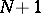(nodes and the value of the coefficient). The parameters are determined by the requirement that (*) is exact for all polynomials of degreeor less, or equivalently, for the monomials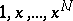. The parameteris obtained from the condition that the quadrature formula is exact for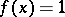, and is equal to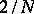. The nodesturn out to be real only forand. Forthe nodes were calculated by P.L. Chebyshev. Foramong the nodes of the Chebyshev quadrature formula there always are complex ones (cf. ). The algebraic degree of precision of the Chebyshev quadrature formula is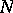for oddandfor even. Formula (*) was proposed by Chebyshev in 1873.